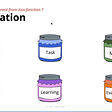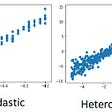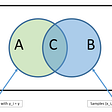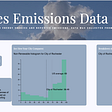# Price bundling using Genetic Algorithm in R

`#Load Customer dataCustomer <- read.csv(file.csv, sep = ",", header = T)#Price <- c(35.0,75.0,64.0,75.0,82.0,92.0,100.0)attach(Customer)library(matrixStats)Func <- function(x){  MarketSur <- cbind(Internet-x,TV-x,Cell.Phone-x,                         Internet+TV-x, Internet+Cell.Phone-x,                         TV+Cell.Phone-x, Internet+TV+Cell.Phone-x)  MaxSur <- rowMaxs(MarketSur)  Prod <- function(MarketSur)  {    s <- dim(MarketSur)    Mt <- vector("numeric", s)    for(i in 1:s){      if(max(MarketSur[i,])<0)      {        Mt[i] <- 0      }      else      {        Mt[i] <-  which.max(MarketSur[i,])      }          }    return(Mt)  }  Bt <- Prod(MarketSur)  Rev <- vector("numeric",77)  #Rev <- x[Bt]  for(i in 1:77){    if(Bt[i] == 0)    {      Rev[i] = 0    }    else{      Rev[i] = x[Bt[i]]    }  }  Pen <-vector("numeric",12)  Pen <- x-x  Pen <- x-x  Pen <- x-x  Pen <- x-x  Pen <- x-x  Pen <- x-x  Pen <- x-x  Pen <- x-x  Pen <- x-x  Pen <- x-x  Pen <- x-x  Pen <- x-x  for(i in 1:12)  {    if(Pen[i]>0)    {      Pen[i] <- Pen[i]    }    else    {      Pen[i] <- 0    }  }  TotalRev <- sum(Rev) - 500*sum(Pen,na.rm=F)  return(TotalRev)}  #GAmodel <- rbga.bin(size = 7, popSize = 200, iters = 100, mutationChance = 0.01,                     #elitism = T, evalFunc = EvalFunc)## calculate the optimum solution using Genetic Algorithm#numvar <- 7#resultGA <- GA(EvalFunc, optimType="MAX", numVar, numPopulation=20,               #maxIter=100, rangeVar, Pm, Pc) L <- c(30,30,30,30,30,30,30)U <- c(100,100,100,100,100,100,100)suggestedValue <-c(50,35,65,71,71,71,85)GA <- ga(type = "real-valued", fitness =  Func,         lower = L, upper = U,         popSize = 2000, maxiter = 500)summary(GA)plot(GA)`

--

--

--

## More from Himanshu Bhardwaj

MBA, IIM Shillong

Love podcasts or audiobooks? Learn on the go with our new app.

## Recipe Of Deep learning## Still Image 0 Video 1## How to Deploy Machine Learning Models to the Cloud Quickly and Easily## Audio classification of Dogs and Cats using CNN and RNN## Combining Bayesian Neural Networks and Ensemble techniques## PROBABILISTIC ALGORITHMS## Scripting for Linux: Image Finder## From Research to Practice## Himanshu Bhardwaj

MBA, IIM Shillong

## ‘Visualizing Emissions and Energy Mix Data’ Final Report## K-Means Clustering Key Points## Examining the relevance of various predictors for Jewelry spent